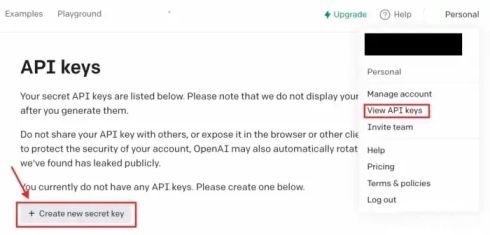0

# 学会开发自己的Python AI应用【OpenAI API篇】

## 生成 API 密钥## 使用 Python接入 OpenAI API

``````pip install openai
``````

## 1.文本生成

Mike：我不喜欢做作业！

Sentiment：

``````import os import openai
openai.api_key = os.getenv("OPENAI_API_KEY") prompt =  """
Decide whether a Mike's sentiment is positive, neutral, or negative.

Mike: I don't like homework!
Sentiment:
"""
response = openai.Completion.create( model="text-davinci-003", prompt=prompt, max_tokens=100, temperature=0  )  print(response)
``````

``````{
"choices": [
{
"finish_reason": "stop",
"index": 0,
"logprobs": null,
"text": "Negative"
}
],
...
}
``````

model ：要使用的模型的 ID（在这里你可以看到所有可用的模型）

Prompt：生成结果的触发指令

max_token：完成时生成的最大token数量（这里可以看到OpenAI使用的tokenizer）

temperature：要使用的采样策略。 接近 1 的值会给模型带来更多风险/创造力，而接近 0 的值会生成明确定义的答案。

## 2. 代码生成

Codex 模型系列是经过自然语言和数十亿行代码训练的 GPT-3 系列的后代。 借助 Codex，我们可以将注释转化为代码、重写代码以提高效率等等。

``````import os
import openai

openai.api_key = os.getenv("OPENAI_API_KEY")

response = openai.Completion.create(
model="code-davinci-002",
prompt="\"\"\"\nCreate an array of weather temperatures for Shanghai\n\"\"\"",
temperature=0,
max_tokens=256,
top_p=1,
frequency_penalty=0,
presence_penalty=0
)

print(response)
``````

``````{
"choices": [
{
"finish_reason": "stop",
"index": 0,
"logprobs": null,
"text": "\n\nimport numpy as np\n\ndef create_temperatures(n):\n    \"\"\"\n    Create an array of weather temperatures for Shanghai\n    \"\"\"\n    temperatures = np.random.uniform(low=14.0, high=20.0, size=n)\n    return temperatures"
}
],
...
}
}
``````

``````import numpy as np

def create_temperatures(n):
temperatures = np.random.uniform(low=14.0, high=20.0, size=n)
return temperatures
``````

## 3. 图像生成

``````import openai

response = openai.Image.create(
prompt="A fluffy white cat with blue eyes sitting in a basket of flowers, looking up adorably at the camera",
n=1,
size="1024x1024"
)
image_url = response['data']['url']
print(image_url)
``````### “学会开发自己的Python AI应用【OpenAI API篇】”的评论:

##### 关于作者##### overfit同步小助手